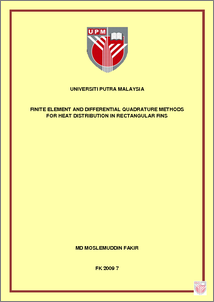# Finite Element and Differential Quadrature Methods for Heat Distribution in Rectangular Fins

## Citation

Fakir, Md. Moslemuddin (2009) Finite Element and Differential Quadrature Methods for Heat Distribution in Rectangular Fins. PhD thesis, Universiti Putra Malaysia.

## Abstract

Presently there are many numerical solution techniques such as finite element method (FEM), differential quadrature method (DQM), finite difference method (FDM), boundary element method (BEM), Raleigh-Ritz method (RRM), etc. These methods have their respective drawbacks. However, FEM and DQM are important techniques among those. The conventional FEM (CFEM) provides flexibility to model complex geometries than FDM and conventional DQM (CDQM) do in spite some of its own drawbacks. It has been widely used in solving structural, mechanical, heat transfer, and fluid dynamics problems as well as problems of other disciplines. It has the characteristic that the solution must be calculated with a large number of mesh points (uniformly distributed) in order to obtain moderately accurate results at the points of interest. Consequently, both the computing time and storage required often prohibit the calculation. Therefore, focus is given to optimize the CFEM. The Optimum FEM (OFEM) has been presented in this thesis to solve heat conduction problems in rectangular thin fins. This method is a simple and direct technique, which can be applied in a large number of cases to circumvent the computational time and complexity. The accuracy of the method depends mainly on the accuracy of the mesh generation (non-uniformly distributed) and stiffness matrix calculation, which is a key of the method. In this thesis, the algorithm for OFEM solution and the optimum mesh generation formula have been developed and presented. The technique has been illustrated with the solution of four heat conduction problems in fins for two types of mesh size distribution (uniformly distributed and non-uniformly distributed). The obtained OFEM results are of good accuracy with the exact solutions. It is also shown that the obtained OFEM results are at least 90% and 7% improved than those of similar published CFEM and ODQM results respectively. This method is a vital alternative to the conventional numerical methods, such as FDM, CFEM and DQM. On the other hand, DQM is suitable for simple geometry and not suitable for practical large-scale problems or on complex geometries. DQM is used efficiently to solve various one-dimensional heat transfer problems. For two-dimensional case, this technique is so far used to solve Poisson’s equation and some fluid flow problems but not the heat conduction problems in fins. Hence, in this thesis, a twodimensional heat conduction problem in a thin rectangular fin is solved using DQM by means of the accurate discretization (for uniformly distributed (CDQM) and non-uniformly distributed (ODQM) mesh size. DQM optimum discretization rule and mesh generation formula have been presented. The governing equations have been discretized according to DQM rule.The technique has been illustrated with the solution of two two-dimentional heat conduction problems in fins. The obtained results show that the DQM results are of good accuracy with the FEM results. Optimum DQM (ODQM) shows better accuracy and stability than CDQM and CFEM. But in some cases, OFEM shows better efficiency than ODQM.Preview
PDF
FK_2009_7a.pdfView Item